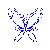## matplotlib.pyplot包来绘制需要的蝴蝶曲线图。先看下漂亮的蝴蝶曲线吧。# 1.首先我们需要确定蝴蝶曲线的函数表达# 2.选择python里面的matplotlib.pyplot作为画图工具

## 1.首先导入python包

import numpy as np
import matplotlib.pyplot as plt


## 2.设置个参数的值

t = np.arange(0.0, 12*np.pi, 0.01)
x = np.sin(t)*(np.e**np.cos(t) - 2*np.cos(4*t)-np.sin(t/12)**5)
y = np.cos(t)*(np.e**np.cos(t) - 2*np.cos(4*t)-np.sin(t/12)**5)


## 3.根据公式，使用numpy里面的函数使用plt画出所需图片

plt.figure(figsize=(8,6))
plt.axis('off')
plt.plot(x,y,color='blue',linewidth = '2')
#plt.show()
plt.savefig("butter.jpg",dpi=400)## 4.使用Image重新调整图片到适当的大小使其符合favicon大小

from PIL import Image
im = Image.open("butter.jpg")
favicon = im.resize((50,50))
favicon.save("favicon.ico")